# Clock

What time is now, if the time elapsed after noon is 2/10 of time that elapses before midnight?

Result

hh:mm = 14:00#### Solution: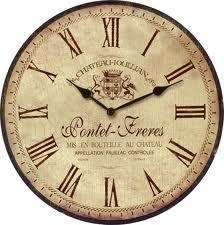We would be very happy if you find an error in the example, spelling mistakes, or inaccuracies, and please send it to us. We thank you!Tips to related online calculators
Need help calculate sum, simplify or multiply fractions? Try our fraction calculator.
Do you have a linear equation or system of equations and looking for its solution? Or do you have quadratic equation?
Do you want to convert time units like minutes to seconds?

## Next similar math problems:

• Pupil ageI am a pupil of the primary school, I spent 1/4 of my age on the exercise of parents with children, 1/3 on drawing, 1/6 on flute. I did not have a ring for the first three years of my life and never went to two rings at once. How old am I?
• Charles Bridge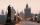Charles Bridge was 15 times older than Frantův bridge from 1900 in 1931. How old is the Charles Bridge?
• Eggs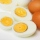One egg boiled in 10 minutes, how long will it take to boil ten eggs at a time?
• Father and son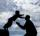Father is 44 years old, his son 16 years. Determine how many years ago was the father five times older than the son.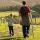Daddy is a 46 year old and son is at age of 16. When (which year) was/will daddy 5 times more years than his son?
• Mixed2improperWrite the mixed number as an improper fraction. 166 2/3
• Unknown numberDetermine the unknown number that is equal to a quarter of the fifth of number, which is by 152 more than unknown number.
• Jam cakes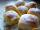Mom baked a third of plum jam cakes, one third cheesecakes and 18 poppy. How many cakes she had bake?
• Equation 20In given equation: 8/9-4/5=2/9+x, find x
• Fraction + eqSolve following simple equation with fractions: -5/6(8+5b) = 75 + 5/3b
• Write 2Write 791 thousandths as fraction in expanded form.
• Missing numberBlank +1/6 =3/2 find the missing number
• Evaluate expressionCalculate the value of the expression z/3 - 2 z/9 + 1/6, for z = 2
• Find unknownFind unknown numerator: 4/8 + _/8 = 1
• Eq1Solve equation: 4(a-3)=3(2a-5)
• Pizza 4Marcus ate half pizza on monday night. He than ate one third of the remaining pizza on Tuesday. Which of the following expressions show how much pizza marcus ate in total?
• Cakes1/3 poppy cake, 1/3 apple, 15 pieces of cheese. How many are totally cakes?